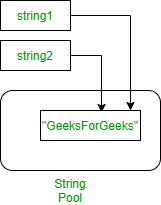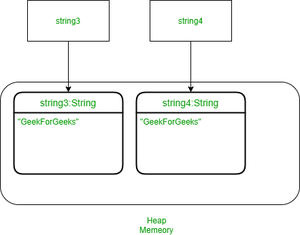# Java String Literals as Constants or Singletons

• Difficulty Level : Medium
• Last Updated : 20 Jul, 2021

String is a sequence of characters, which is widely used in Java Programming. In the Java programming language, strings are objects. In Java, the virtual machine may only create a single String instance in memory, if we use the same string in other string variable declarations. Let us consider illustrations for the sake of understanding which is as follows:

Illustration 1:

```String string1 = "GeeksForGeeks";
String string2 = "GeeksForGeeks";```

In the above example, the java virtual machine will create only one instance of “GeeksForGeeks” in memory. There are two different variables, initialized to “GeeksForGeeks” string will point to the same String instance in memory. The figure below depicts example more accurately:-The string literal thus becomes a de facto constant or singleton. More precisely in java, objects representing Java String literals are obtained from a constant String pool which is internally kept by a java virtual machine. This means, that even classes from different projects compiled separately, but which are used in the same application may share constant String objects. The sharing happens at runtime and hence it is not a compile-time feature.

Illustration 2: If we want those two string variables point to separate String objects then we use the new operator as follows:

```String string1 = new String("GeeksForGeeks");
String string2 = new String("GeeksForGeeks");```

The above code will create two different memory objects in memory to represent them even if the value of two strings is the same. The image below depicts the use of new operator:Note: From the image above it is clearly depicted that two different memory blocks are created for two different string which contains same value string.

Example 1:

## Java

 `// Java Program to Illustrate String Literals as ` `// Constants or Singletons ` ` `  `// Main class ` `// StringSingleton ` `public` `class` `GFG { ` ` `  `    ``// Main driver method ` `    ``public` `static` `void` `main(String[] args) ` `    ``{ ` ` `  `        ``// Strings ` `        ``String string1 = ``"GeeksForGeeks"``; ` `        ``String string2 = ``"GeeksForGeeks"``; ` `        ``String string3 = ``new` `String(``"GeeksForGeeks"``); ` `        ``String string4 = ``new` `String(``"GeeksForGeeks"``); ` ` `  `        ``// Checking if two string are same or not ` `        ``if` `(string1 == string2) { ` ` `  `            ``// Returns true ` `            ``System.out.println(``"True"``); ` `        ``} ` `        ``else` `{ ` ` `  `            ``// Returns false ` `            ``System.out.println(``"False"``); ` `        ``} ` ` `  `        ``// Again checking if two string are same or not ` `        ``if` `(string1 == string3) { ` ` `  `            ``// Returns false ` `            ``System.out.println(``"True"``); ` `        ``} ` `        ``else` `{ ` ` `  `            ``System.out.println(``"False"``); ` `        ``} ` ` `  `        ``// Again checking if two string are same or not ` `        ``if` `(string4 == string3) { ` ` `  `            ``// Returns false ` `            ``System.out.println(``"True"``); ` `        ``} ` `        ``else` `{ ` `            ``System.out.println(``"False"``); ` `        ``} ` `    ``} ` `}`

Output

```True
False
False```

In the above program, a total of 3 objects will be created. Now, if we want to compare 2 string contents, we can use the equals method, and ‘==’ operator can be used to compare whether two string references are the same or not.

Example 2:

## Java

 `// Java Program to Illustrate String Literals as ` `// Constants or Singletons ` ` `  `// Main class ` `// StringSingleton ` `public` `class` `GFG { ` ` `  `    ``// Main driver method ` `    ``public` `static` `void` `main(String[] args) ` `    ``{ ` `        ``// Strings ` `        ``String string1 = ``"GeeksForGeeks"``; ` `        ``String string3 = ``new` `String(``"GeeksForGeeks"``); ` ` `  `        ``// Print and display statements ` ` `  `        ``// Displays true ` `        ``System.out.println(string1.equals(string3)); ` ` `  `        ``// Displays false ` `        ``System.out.println(string1 == string3); ` `    ``} ` `}`

Output

```true
false```

My Personal Notes arrow_drop_up
Recommended Articles
Page :